# What is the marginal distribution in statistics

## Marginal distribution / marginal distribution

Your multidimensional random variable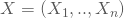can be achieved by jointly distributing thedescribe. You designate the distribution of an individual variable as marginal or marginal distribution if the realizations of the other variables are not taken into account.

You can again distinguish the discrete from the continuous case:

Discrete case:

For example, you have the two-dimensional probability function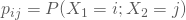for German grades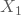of boys and girls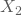determined as follows: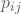: Gender 1: girl 2: boys total: German grade 1 0,061 0,030 0,091 2 0,273 0,152 0,424 3 0,182 0,212 0,394 4 0,030 0,061 0,091 total 0,545 0,455 1

Inside the table are the, with which an arbitrarily chosen student has the German grade i and the gender j: so is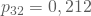the probability with which a randomly selected student has achieved a German grade of 3 and is male.

The line sums then give the probabilities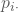with which a randomly selected student achieved the respective German grade: This is the marginal or marginal distribution of.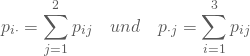The marginal distribution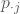of, i.e. the column totals, tell you the probability of a randomly selected student being female or male.

Do you consider the distribution function instead of the probability function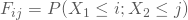, so you get the marginal distribution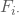the probability with which a student will achieve at least the grade i.

Constant fall:

In the continuous case you have given a common density function f (X, Y) for two random variables X and Y.

You get the density function for X without taking into account the realizations of Y, by integrating over all possible realizations of Y: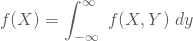and the one for Y by integrating over the domain of X: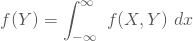If you do not consider the density function but the distribution function F (X, Y), you get the marginal distribution of Y without considering X as the probability with which Y is less than or equal to y.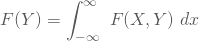In the case of a more than two-dimensional distribution, you get the marginal distributions of the kth individual variables by integrating over all other variables.